UnboundEd Mathematics Guide

# Operations & Algebraic Thinking: Unbound A Guide to Grade 4 Mathematics Standards

?
What's in a Content Guide and how do I use it?
Get answers to all your Content Guide questions, including what's in each part and how they can be used in your role at your school
View FAQs

4.OA.A | Use the four operations with whole numbers to solve problems.

4.OA.B | Gain familiarity with factors and multiples.

4.OA.C | Generate and analyze patterns.

4.MD.A | Solve problems involving measurement and conversion of measurements.

Welcome to the UnboundEd Mathematics Guide series! These guides are designed to explain what new, high standards for mathematics say about what students should learn in each grade, and what they mean for curriculum and instruction. This guide, the first for Grade 4, includes three parts. The first part gives a “tour” of the standards in the Operations & Algebraic Thinking domain using freely available online resources that you can use or adapt for your class. The second part shows how Operations & Algebraic Thinking relates to other concepts in Grade 4. And the third part explains the progression of learning for multiplication and division of whole numbers in Grades K-5. Throughout all of our guides, we include a large number of sample math problems. We strongly suggest tackling these problems yourself to help best understand the methods and strategies we’re covering, and the potential challenges your students might face.

# Part 1: What do the standards say?

In Grade 4, the Operations & Algebraic Thinking (OA) domain describes some of the important expectations around multiplication and division and problem-solving. The domain is composed of three clusters and each cluster has associated standards.

The first cluster in the OA domain is part of the major work of Grade 4, as indicated by the green square.1 The Common Core State Standards for Mathematics (CCSSM) are organized into major, additional and supporting clusters in the Focus by Grade Level documents from Student Achievement Partners. The Common Core State Standards for Mathematics (CCSSM) are organized into major, additional and supporting clusters in the Focus by Grade Level documents from Student Achievement Partners. The Common Core State Standards for Mathematics (CCSSM) are organized into major, additional and supporting clusters in the Focus by Grade Level documents from Student Achievement Partners. Teachers should spend a large majority of their time teaching major clusters. The second cluster is a supporting cluster (blue square), and the third cluster is an additional cluster (yellow square). Supporting clusters enhance the focus and coherence of the major clusters, and additional clusters are important but do not carry as much instructional emphasis as the major work. While we will focus on the OA clusters in this guide, it’s important to note that the clusters in the Number & Operations in Base Ten (NBT) domain are tightly intertwined with the OA clusters. The NBT standards describe the place value strategies and articulate the size of the numbers being multiplied and divided in word problems. It’s likely that a carefully sequenced curriculum map will begin with both OA and NBT standards, as the skills and ideas related to multiplication and multiplicative comparison described in these two domains play a role in many other parts of Grade 4. However, since the NBT standards are fairly extensive, we will discuss them in a separate content guide.

The other cluster discussed in this guide comes from the Measurement & Data domain. This is a supporting cluster, and the standards in this cluster can help students deepen their understanding of the standards in the OA clusters. We will talk about these standards in detail in Part 2.

It’s important to note that the clusters, and the standards within the clusters, are not necessarily sequenced in the order in which they have to be taught. (Standards are only a set of expectations of what students should know and be able to do by the end of each year; they don’t prescribe an exact sequence or curriculum.) So planning your instruction sequence carefully can ensure your students continue to build on previous understandings. As we go, think about the connections you see between standards, and how you can use these connections to help students build on their previous understandings.

Throughout the guide, we’ll look at many examples of tasks and lessons that focus on students’ abilities to make sense of problems and persevere in solving them (MP.1). As students are exposed to varied contexts and problem types, they will need to think carefully to understand each problem and develop an appropriate solution method.

The first cluster in the Operations & Algebraic Thinking domain has three standards and is about multiplicative comparison and problem-solving. Let’s begin by reading these standards, and then we’ll think through what they mean and how they look in practice.

4.OA.A | Use the four operations with whole numbers to solve problems.

 4.OA.A.1 Interpret a multiplication equation as a comparison, e.g., interpret 35 = 5 × 7 as a statement that 35 is 5 times as many as 7 and 7 times as many as 5. Represent verbal statements of multiplicative comparisons as multiplication equations. 4.OA.A.2 Multiply or divide to solve word problems involving multiplicative comparison, e.g., by using drawings and equations with a symbol for the unknown number to represent the problem, distinguishing multiplicative comparison from additive comparison.* 4.OA.A.3 Solve multistep word problems posed with whole numbers and having whole-number answers using the four operations, including problems in which remainders must be interpreted. Represent these problems using equations with a letter standing for the unknown quantity. Assess the reasonableness of answers using mental computation and estimation strategies including rounding. *See Glossary, Table 2.

## Multiplicative Comparison

In Grade 4, students continue their study of multiplication and division of whole numbers. They continue to work with multiplication and division situations involving equal groups, arrays, and area, which were emphasized in Grade 3. ( 3.OA.A.3, 3.MD.C.7) However, new to Grade 4 is the concept of multiplicative comparison. (4.OA.A.1 )

Multiplicative comparison involves comparing quantities multiplicatively instead of additively, something students have been working on since Grade 1. (1.OA.A.1)

 Multiplicative comparison “My friend has 2 apples and I have 10 apples, so I have 5 times as many apples as she does.” 2 × ___ = 10 Additive comparison “My friend has 2 apples and I have 10 apples, so I have 8 more apples than she does.” 2 + ___ = 10

In additive comparisons, the underlying question is: What amount would be added to one quantity in order to result in another? By contrast, in multiplicative comparisons, the underlying question is: What factor would multiply one quantity in order to result in the other?2 Progressions for the Common Core State Standards in Mathematics (draft): K, Counting and Cardinality; K–5, Operations and Algebraic Thinking, p. 29. Progressions for the Common Core State Standards in Mathematics (draft): K, Counting and Cardinality; K–5, Operations and Algebraic Thinking, p. 29. Progressions for the Common Core State Standards in Mathematics (draft): K, Counting and Cardinality; K–5, Operations and Algebraic Thinking, p. 29.

Students learn to make the distinction between multiplicative and additive comparisons through lots of practice. They should continue to build on their experience with additive comparison problems (e.g., with multidigit whole numbers (4.NBT.B.)4). Similarly, they should engage extensively with multiplicative comparison word problems where the unknown is all positions. (4.OA.A.2 )

With respect to their previous experiences with multiplication, students should understand that multiplicative comparison is different from equal groups multiplication. In addition to understanding that 3 x 6 = 18 can be interpreted as “3 groups of 6 is equal to 18,” students can also interpret the equation as 18 is 3 times as many as 6 or 6 times as many as 3. (4.OA.A.1)

Multiplication and division problem situations

The table below shows the different multiplication and division problem situations students should master in Grades 3-5. Of particular importance in Grade 4 is the “compare” row; the three subtypes describe multiplicative comparison situations. It will be helpful to spend some time digging into all of the different problem situations shown in the table, as we will refer to them extensively throughout the rest of this discussion.

Many of us have learned that solving word problems involves finding “key words,”— words like “more” and “total” tell us to add, while words like “fewer” and “less” tell us to subtract. But what about a problem like this:

Lucy has six fewer apples than Julie. Lucy has eight apples. How many apples does Julie have?3 Adapted from CCSSM Table 2. Adapted from CCSSM Table 2. Adapted from CCSSM Table 2.

The “key word” in this problem, “fewer,” actually hints at the wrong operation; subtracting will not result in the correct answer.

A better way to help students with problem-solving is to help them think situationally about the varied contexts. Using word problems to create meaning for operations helps students to better understand how to apply operations.

Common multiplication and division situations (1)

 UNKNOWN PRODUCT GROUP SIZE UNKNOWN (“HOW MANY IN EACH GROUP?” DIVISION) NUMBER OF GROUPS UNKNOWN (“HOW MANY GROUPS?” DIVISION) 3 x 6 = ? 3 x ? = 18, and 18 ÷ 3 = ? ? x 6 = 18, and 18 ÷ 6 = ? EQUAL GROUPS There are 3 bags with 6 plums in each bag. How many plums are there in all? Measurement example. You need 3 lengths of string, each 6 inches long. How much string will you need altogether? If 18 plums are shared equally into 3 bags, then how many plums will be in each bag? Measurement example. You have 18 inches of string, which you will cut into 3 equal pieces. How long will each piece of string be? If 18 plums are to be packed 6 to a bag, then how many bags are needed? Measurement example. You have 18 inches of string, which you will cut into pieces that are 6 inches long. How many pieces of string will you have? ARRAYS (2), AREA (3) There are 3 rows of apples with 6 apples in each row. How many apples are there? Area example. What is the area of a 3 cm by 6 cm rectangle? If 18 apples are arranged into 3 equal rows, how many apples will be in each row? Area example.A rectangle has area 18 square centimeters. If one side is 3 cm long, how long is a side next to it? If 18 apples are arranged into equal rows of 6 apples, how many rows will there be? Area example. A rectangle has area 18 square centimeters. If one side is 6 cm long, how long is a side next to it? COMPARE A blue hat costs \$6. A red hat costs 3 times as much as the blue hat. How much does the red hat cost? Measurement example. A rubber band is 6 cm long. How long will the rubber band be when it is stretched to be 3 times as long? A red hat costs \$18 and that is 3 times as much as a blue hat costs. How much does a blue hat cost? Measurement example. A rubber band is stretched to be 18 cm long and that is 3 times as long as it was at first. How long was the rubber band at first? A red hat costs \$18 and a blue hat costs \$6. How many times as much does the red hat cost as the blue hat? Measurement example. A rubber band was 6 cm long at first. Now it is stretched to be 18 cm long. How many times as long is the rubber band now as it was at first? GENERAL a x b = ? a x ? = p and p ÷ a = ? ? x b = p, and p ÷ b = ?

(1) The language in the array examples shows the easiest form of array problems. A harder form is to use the terms rows and columns: The apples in the grocery window are in 3 rows and 6 columns. How many apples are in there? Both forms are valuable.

(2) Area involves arrays of squares that have been pushed together so that there are no gaps or overlaps, so array problems include these especially important measurement situations.

(3) The first examples in each cell are examples of discrete things. These are easier for students and should be given before the measurement examples.

Source: CCSSM Table 2

In Grade 4, students use multiplication and division to solve word problems in situations involving multiplicative comparison.4.OA.A.2 These situations are described in the third row of Table 2; take a moment to focus on those subtypes, as they are at the heart of our work in Grade 4. Let’s take a closer look at them now.

### Compare problems

The following task shows an example of each of the compare problem subtypes described in the table above. The unknown is in a different position in each problem.

#### Comparing Money Raised

1. Helen raised \$12 for the food bank last year and she raised 6 times as much money this year. How much money did she raise this year?

1. Sandra raised \$15 for the PTA and Nita raised \$45. How many times as much money did Nita raise as compared to Sandra?

1. Luis raised \$45 for the animal shelter, which was 3 times as much money as Anthony raised. How much money did Anthony raise?

Solution

Solution: Tape diagram

1. She raised six times as much money (as shown in the diagram) so she raised 6 × 12 = 72.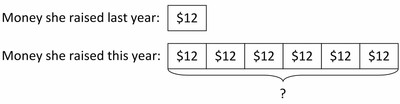Helen raised \$72 this year.

1. ? × 15 = 45 is equivalent to 45 ÷ 15 = ?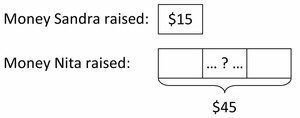Nita raised 3 times as much as Sandra.

1. 3 × ? = 45 is equivalent to 45 ÷ 3 = ?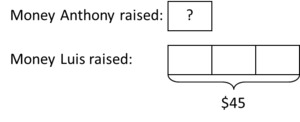Anthony raised \$15.

Solution: Writing multiplication equations for division problems

1. Helen raised 6 × \$12 this year, so she raised \$72 this year.
2. This is a “Number of Groups Unknown” problem. We can represent the question as

? × 15 = 45

or

45 ÷ 15 = ?

So Nita raised 3 times as much money as Sandra.

1. This is a “Group Size Unknown” problem. We can represent the question as

3 × ? = 45

or

45 ÷ 3 = ?

So Anthony raised \$15.

In the first problem, the product is unknown; students need to multiply 12 by 6 to find the total. In the second problem, the multiplier (or “scale factor”) is unknown; students can use division to solve this problem (45 ÷ 15 = ?) or they can think of this as an unknown factor problem (15 × ? = 45). In the third problem, the smaller amount is unknown; students can use division to solve this problem (45 ÷ 3 = ?) or they can think of this as an unknown factor problem (3 × ? = 45). (3.OA.B.6) As with additive comparison problems, using tape diagrams with multiplicative comparisons is a helpful tool for problem-solving. The sample solutions in this task illustrate how tape diagrams can be used to represent compare situations. Each of the tape diagrams in the solution is accompanied by an equation with a symbol for the unknown (e.g., a box or question mark). Using a symbol to represent the unknown in an equation is an expectation in Grade 4 (4.OA.A.2) and has been an expectation since first grade. (1.OA.A.1)

### Equal groups, arrays, and area

#### Grade 4, Module 3, Lesson 11: Problem Set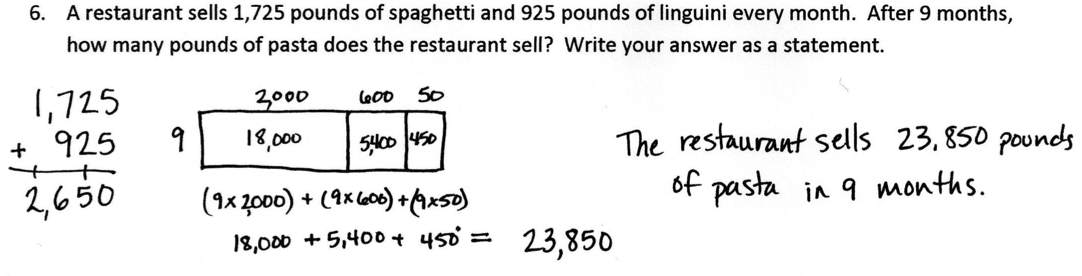While we might be tempted to push students to use the standard algorithm to multiply, this is not required in Grade 4; we want to ensure that students spend significant time building conceptual understanding of multiplication. Fluency with the standard algorithm for multiplication is not required until the end of Grade 5, (5.NBT.B.5) and not until the end of Grade 6 for division. (6.NS.B.2)

## Using the four operations to solve problems

The following examples illustrate different kinds of multistep word problems. In the first example, students are expected to solve a single problem that involves several steps in order to determine the total amount of money earned for both days.

#### Grade 3, Module 3, Lesson 7: Application Problem

The basketball team is selling T-shirts for \$9 each. On Monday, they sold 4 T-shirts. On Tuesday, they sold 5 times as many T-shirts as on Monday. How much money did the team earn altogether on Monday and Tuesday?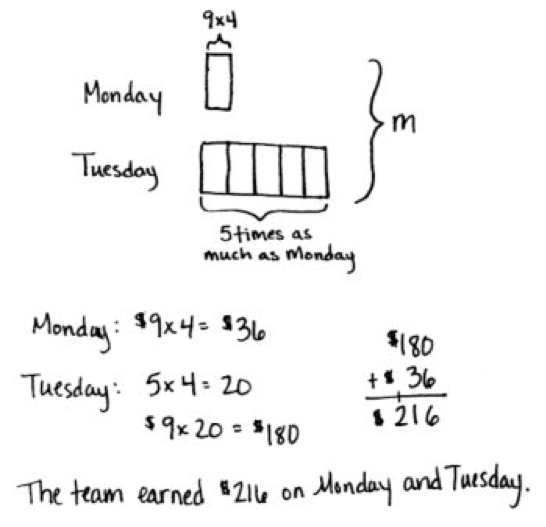Note: This is a multi-step word problem reviewing multiplying by multiples of 10 from Lesson 5, including multiplicative comparison.

This solution is modeled using different kinds of representations. The step involving multiplicative comparison is modeled with a tape diagram and labeled equations are used to show the multiplication. Problems like this also give opportunities for students to assess the reasonableness of answers using mental computation and estimation strategies, including rounding. (4.OA.A.3) We might ask, “Is \$216 a reasonable answer? Why?” A student might respond using language that reflects the following reasoning:

• Each t-shirt cost about \$10. If the team sold a total of 24 t-shirts, then the team earned about \$240 because \$10 x 24 = \$240. My answer is reasonable, because \$216 is close to, but less than \$240.

In order to think about reasonableness, students need to have a solid understanding of the meaning of operations and of estimation strategies; also, rounding can play a key role in estimating. Students begin rounding in Grade 3, to the nearest 10 and 100, based on place value understanding (e.g., using the number line). (3.NBT.A.1) They build on this understanding in Grade 4 to round multi-digit whole numbers to any place. (4.NBT.A.3 )Asking questions like the one above, and building students’ abilities to answer them thoughtfully, should be a regular part of instruction in Grade 4.

While the prior example was a single, extended task, multistep problems can also be comprised of a series of questions developing from a particular context, as in the next example. Students have to pay close attention to the language in each part to determine whether the relationship being asked about is additive or multiplicative. Given that there are so many questions in this task, the size of the numbers is restricted.

#### Carnival Tickets

Every year a carnival comes to Hallie's town. The price of tickets to ride the rides has gone up every year.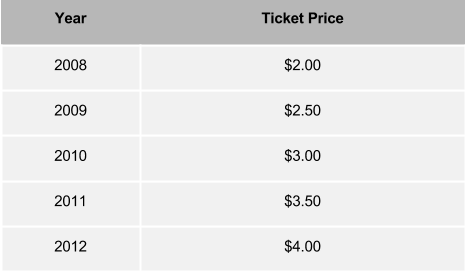1. In 2008, Hallie’s allowance was \$9.00 a month. How many carnival tickets could she buy with one month's allowance?

1. If her allowance had stayed the same, \$9.00 a month, how many carnival tickets could she buy in 2012?

1. In 2012, Hallie’s allowance was \$14.00 per month. How much did her monthly allowance increase between 2008 and 2012?

1. How much more did a carnival ticket cost in 2012 than it did in 2008?

1. Was Hallie able to buy more carnival tickets in 2008 or in 2012 with one month's allowance?

1. What would Hallie's allowance need to be in 2012 in order for her to be able to buy as many carnival tickets as she could in 2008?

1. What happens to your ability to buy things if prices increase and your allowance doesn’t increase?

HideShow

In part a, students have to interpret the meaning of a remainder, which is a new understanding in Grade 4. (4.OA.A.3) It’s important to emphasize that we want students to be able to interpret the remainder, rather than simply compute quotients that happen to have remainders. In fact, the quotient and remainder can play different roles in the solution to problems:

• In the case of part a above, the answer to the question is the whole number quotient (i.e., the answer is 4 and the quotient is 4 with a remainder of 1).
• In other situations, the remainder might indicate that the answer to the question is different from the whole number quotient (e.g., Each table has 2 chairs. What is the minimum number of tables that are needed to seat 9 students?).
• Further, the remainder might be the answer to the question (e.g., Charlie divided 9 pencils equally into 2 containers so that each container had the maximum number of pencils. How many pencils were left over?).

Remainders have a variety of meaning in the real world, and for students to be successful with interpreting remainders in different situations, it’s important that they have significant experience solving problems for which the remainder takes on varied meanings in context.

It is worth pointing out here that using the common notation (9 ÷ 2 = 4 R 1) for representing remainders in the division of whole numbers is not precise and is inconsistent with the meaning of the equal sign. For example, if 9 ÷ 2 = 4 R 1 and 13 ÷ 3 = 4 R 1, then students might reason that 9 ÷ 2 = 13 ÷ 3, which is not true. A more precise way to show the result of division in terms of a whole number quotient and a remainder is: 9 = 4 x 2 + 1.7 Front Matter for Progressions for the Common Core State Standards in Mathematics (draft), p. 12. Front Matter for Progressions for the Common Core State Standards in Mathematics (draft), p. 12. Front Matter for Progressions for the Common Core State Standards in Mathematics (draft), p. 12. You will likely encounter many resources that use “R” notation; it might be worth a discussion with students about the precision of such notation.

Also, as part of 4.OA.A.3, students should use letters to represent the unknown. This is slightly different from the language in standard 4.OA.A.2, which is about using a “symbol” for the unknown. A letter for an unknown is more formal use of algebraic language that begins in Grade 3 with use of a letter for unknown in two-step problems. (3.OA.D.8) In the example below, students use the letter m to model the number of marbles Presley has.

#### Grade 4, Module 3 Lesson 12: Concept Development, Problem 3

Jayden has 347 marbles. Elvis has 4 times as many as Jayden. Presley has 799 fewer than Elvis. How many marbles does Presley have?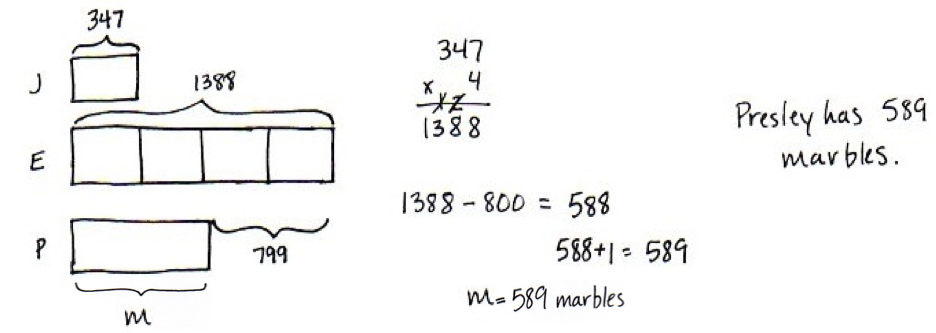In later grades, students will be expected to regularly represent and solve multistep problems, and using a letter for the unknown should be second nature. In Grade 4, we can ensure students are ready for this later work by consistently giving students opportunities to use letters for unknown quantities.

## Factors and Multiples

The second cluster in the Operations & Algebraic Thinking domain is a supporting cluster and deals with factors and multiples. Let’s read the standard associated with this cluster, and then we’ll think through what it means and how it looks in practice.

4.OA.B | Gain familiarity with factors and multiples.

 4.OA.B.4 Find all factor pairs for a whole number in the range 1-100. Recognize that a whole number is a multiple of each of its factors. Determine whether a given whole number in the range 1-100 is a multiple of a given one-digit number. Determine whether a given whole number in the range 1-100 is prime or composite.

### A quick look at decomposition

Factor pairs are a sort of decomposition of numbers; for example, 10 can be decomposed into 2 x 5 or 1 x 10. In order to best support students with this in Grade 4, let’s take a quick look at the roots of decomposition.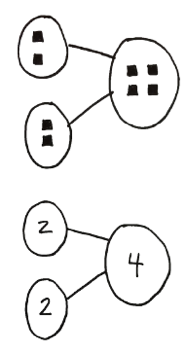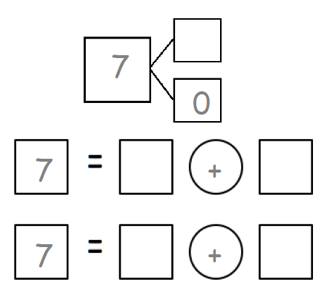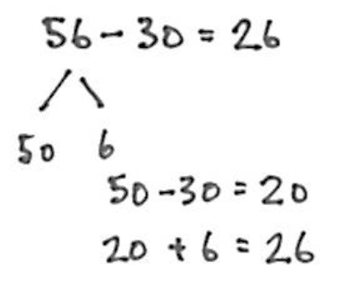• In the first example (Kindergarten), students understand that the number 4 is composed of embedded numbers (2 and 2).
• In the second example (Grade 1), decomposing 7 is related to addition.
• In the third example (Grade 2), 56 is decomposed to make an easier subtraction problem.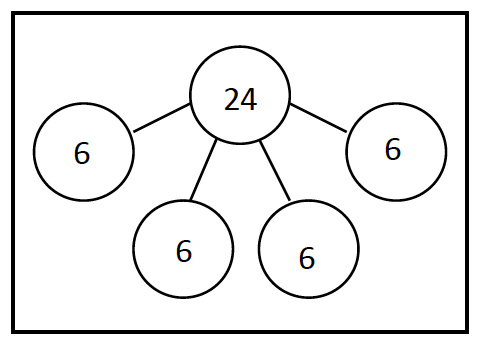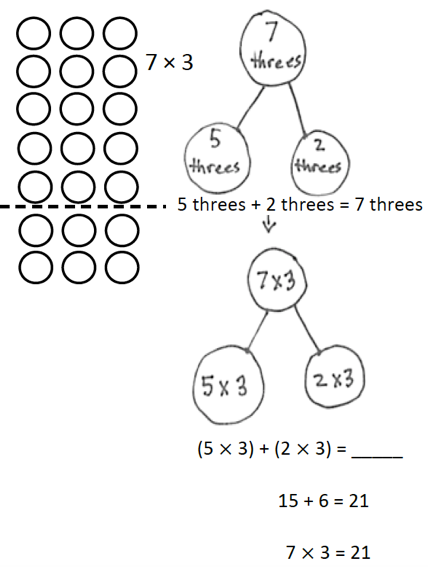• In the fourth example (Grade 3), decomposition is related to equal groups as 24 is decomposed into four sixes (i.e., 6 + 6 + 6 + 6 = 24).
• In the last example (Grade 3), decomposing is connected to the distributive property.

We can address factoring pairs in Grade 4 by using additive decomposition as a starting place; for example, by asking students to decompose a number, like 10, additively prior to introducing factors. Using visual models, such as number bonds, to teach this may be helpful as well.

### Factor pairs as decomposition in Grade 4

In Grade 4, students build on the idea that decomposition can be multiplicative: a number can be multiplicatively decomposed into equal groups and expressed as the product of these factors (called factor pairs).9 Progressions for the Common Core State Standards in Mathematics (draft): K, Counting and Cardinality; K–5, Operations and Algebraic Thinking, p. 30. Progressions for the Common Core State Standards in Mathematics (draft): K, Counting and Cardinality; K–5, Operations and Algebraic Thinking, p. 30. Progressions for the Common Core State Standards in Mathematics (draft): K, Counting and Cardinality; K–5, Operations and Algebraic Thinking, p. 30. Students had some experience with multiplicative decomposition in Grade 3 when applying the associative property of multiplication. (3.OA.B.5 )Students decomposed one factor into a factor pair in order to make an easier multiplication problem (e.g., when multiplying 16 x 3, a student might decompose 16 into 8 x 2 to get (8 x 2) x 3 = 8 x (2 x 3) = 8 x 6 = 48). Now in Grade 4, students find factor pairs for whole numbers within 100 and learn that a prime number has only 1 and itself as factors. By contrast, if a number is not prime (having more than 1 and itself as factors), it is composite. The example below asks students to find factor pairs and determine whether a number is prime or composite.

#### Grade 4, Module 3, Lesson 22: Exit Ticket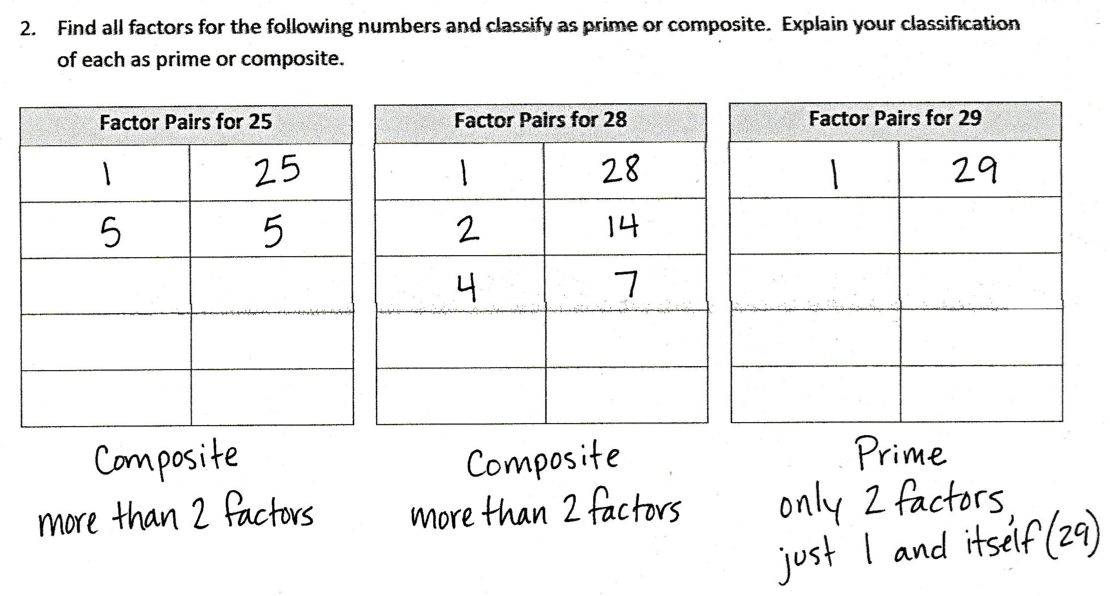While factor is not a new term in Grade 4, the term “multiples” may be new. A whole number is a multiple of each of its factors. Using the example above, 28 is a multiple of 2 and of 14 because 28 = 2 x 14. Precision of mathematical language is important, and we should be attentive to correct usage in instruction. However, the focus for students is on understanding the mathematical concepts of factors and multiples rather than memorizing definitions.

So how does the study of factor pairs and multiples support thinking about multiplication and division? One way is that exploring patterns with multiples of numbers and with factor pairs gives insight into patterns of divisibility. While patterns of divisibility are not required by the standards, they may be helpful strategies for dividing. Students should explore these patterns in the multiplication table rather than memorizing a set of rules. In the following task, students explore patterns with multiples in the multiplication table.

#### Identifying Multiples

Below is a multiplication table for single digit numbers:Use a different copy of the multiplication table for each of the questions below:

1. Color all boxes with multiples of 2 in the multiplication table. What pattern do you see in the colored boxes?

1. Color all boxes with multiples of 3 in the table. How does the pattern of multiples of 3 compare to the pattern of multiples of 2?

1. Color all boxes with multiples of 4 in the table. How is this different from the patterns in (a) and (b)? Why?

HideShow

In this task, students observe that multiplies of 2 are even. Also, the product of a factor pair is a multiple of 3 if one of the factors is divisible by 3 (e.g., 6 x 8 = 48 so 48 is a multiple of 3 because 6 is divisible by 3). Additionally, since 4 is a composite number (i.e., it has another factor other than 1 and 4), the product of a factor pair might be a multiple of 4 even if neither of the factors is divisible by 4 (e.g., 6 x 6 = 36 and 36 is a multiple of 4 even though both factors (6 and 6) are not divisible by 4). This is because both factors are divisible by 2 and 4 has a factor pair of 2 x 2 (e.g., 6 x 6 = (3 x 2) x (3 x 2) = (2 x 2) x (3 x 3) = 4 x 9).

As previously mentioned, the study of factor pairs and multiples also supports application of the associative property of multiplication. In the following example, students use the associative property of multiplication to show that if 6 is a factor of a number, then 2 and 3 are also factors of that number.

#### Grade 4, Module 3, Lesson 23: Concept Development

T: We used the associative property to show that both 2 and 3 are factors of 54.

T: Let’s test this method to see if it works with a number other than 54. Forty-two is 6 times…?

S: 7.

T: Let’s use the associative property to see if 2 and 3 are also factors of 42.

T: (Write 42 = 6 × 7.) How will we rewrite 6?

S: 2 × 3.

T: (Beneath 6 × 7, write = (2 × 3) × 7.) Let’s now move the parentheses to first multiply 3 times 7, to associate 3 with 7 rather than 2. 3 times 7 is?

S: 21.

T: Find the product of 2 and 21. (Pause.) Is it true that 2 times 21 equals 42?

S: Yes!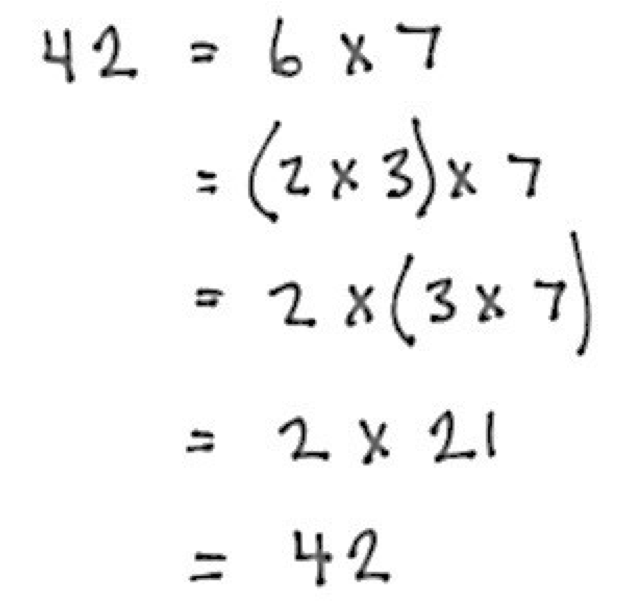Record the thought process as shown to the right. Have students use the associative property to prove that since 6 is a factor of 60, both 2 and 3 are also factors.

Generating and analyzing patterns

The third cluster in the Operations & Algebraic Thinking domain is an additional cluster and deals with generating and analyzing patterns. Let’s read the standard associated with this cluster, and then we’ll think through what it means and how it looks in practice.

4.OA.C | Generate and analyze patterns.

 4.OA.C.5 Generate a number or shape pattern that follows a given rule. Identify apparent features of the pattern that were not explicit in the rule itself. For example, given the rule "Add 3" and the starting number 1, generate terms in the resulting sequence and observe that the terms appear to alternate between odd and even numbers. Explain informally why the numbers will continue to alternate in this way.

This standard has to do with reasoning about number and shape patterns that follow a given rule. Grade appropriate shape patterns might include a repeated sequence of shapes or a growing shape pattern. Number patterns might include repeatedly adding the same whole number or multiplying by the same whole number. In the following task, students have to employ a rule and then make observations about the resulting pattern to answer questions about operations involving even and odd numbers.

#### Doubles Plus One

1. The table below shows a list of numbers. For every number listed in the table, multiply it by 2 and add 1. Record the result on the right.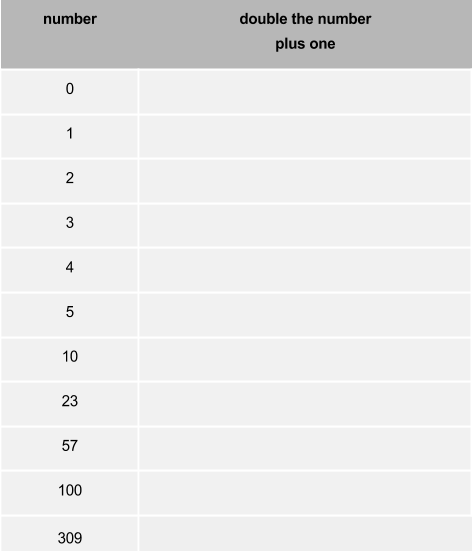1. What do you notice about the numbers you entered into the table?

1. Sherri noticed that all the numbers she entered are odd.
1. Does an even number multiplied by 2 result in an even or odd number? Why do you think this is?

1. Does an odd number multiplied by 2 result in an even or odd number? Why do you think this is?

1. Does an even number plus 1 result in an even or odd number? Why do you think this is?

1. Does an odd number plus 1 result in an even or odd number? Why do you think this is?

1. Explain why the numbers you entered in the table are all odd.

HideShow

Note the emphasis on making conjectures (as in parts i.-iv.) and reasoning (part v.).

The standard states that a rule is given. Students should not have to infer or guess the underlying rule for a pattern. Rather, students should generate a pattern from a given rule and identify features of the given pattern. Traditional pattern activities that ask students to continue a pattern determined by guessing the rule are not a mathematical topic and are not part of the standards.10 Progressions for the Common Core State Standards in Mathematics (draft): Grade 8, High School, Functions, p. 4. Progressions for the Common Core State Standards in Mathematics (draft): Grade 8, High School, Functions, p. 4. Progressions for the Common Core State Standards in Mathematics (draft): Grade 8, High School, Functions, p. 4.

# Part 2: How do Operations & Algebraic Thinking relate to other parts of Grade 4?

There are many connections among standards in Grade 4; if you think about the standards long enough, you’ll probably start to see these relationships everywhere.11 The idea that standards relate strongly to one another is known as coherence, and is a distinctive feature of the Common Core State Standards for Math. If you’re interested in exploring more of the connections between standards, you might want to check out the Student Achievement Partners Coherence Map, which illustrates them visually. The idea that standards relate strongly to one another is known as coherence, and is a distinctive feature of the Common Core State Standards for Math. If you’re interested in exploring more of the connections between standards, you might want to check out the Student Achievement Partners Coherence Map, which illustrates them visually. The idea that standards relate strongly to one another is known as coherence, and is a distinctive feature of the Common Core State Standards for Math. If you’re interested in exploring more of the connections between standards, you might want to check out the Student Achievement Partners Coherence Map, which illustrates them visually. In this section, we’ll talk about the connection between the standards in the Operations & Algebraic Thinking (OA) domain and the standards in the first cluster of the Measurement & Data (MD) domain. The standards in this MD cluster are supporting standards and can be used to support work with the OA standards. As previously mentioned, the OA standards are also closely connected to place value concepts in the Number & Operation in Base ten (NBT) domain. Outside of the connections mentioned in Part 1, we will reserve a more in depth discussion about these connections for another guide. Before talking about the connections to the MD standards in cluster 4.MD.A, let’s read through each of the standards.

4.MD.A | Solve problems involving measurement and conversion of measurements.

 4.MD.A.1 Know relative sizes of measurement units within one system of units including km, m, cm; kg, g; lb, oz.; l, ml; hr, min, sec. Within a single system of measurement, express measurements in a larger unit in terms of a smaller unit. Record measurement equivalents in a two-column table. For example, know that 1 ft is 12 times as long as 1 in. Express the length of a 4 ft snake as 48 in. Generate a conversion table for feet and inches listing the number pairs (1, 12), (2, 24), (3, 36), ... 4.MD.A.2 Use the four operations to solve word problems involving distances, intervals of time, liquid volumes, masses of objects, and money, including problems involving simple fractions or decimals, and problems that require expressing measurements given in a larger unit in terms of a smaller unit. Represent measurement quantities using diagrams such as number line diagrams that feature a measurement scale. 4.MD.A.3 Apply the area and perimeter formulas for rectangles in real world and mathematical problems. For example, find the width of a rectangular room given the area of the flooring and the length, by viewing the area formula as a multiplication equation with an unknown factor.

## Measurement problems and conversions

Students use measurement contexts to support adding, subtracting, multiplying, and dividing with whole numbers as well as with simple fractions and decimals. (4.MD.A.2) It’s important that they solve word problems related to measurement contexts that theyre familiar with. In Grade 4, these include distance, intervals of time, liquid volume, mass of objects, and money. More specifically, appropriate measurement contexts include length (inches, feet, centimeters, and meters), time, and money (whole number quantities like \$3, 5 dimes, 13 cents), which students learned in Grade 2. ( 2.MD.B.5, 2.MD.C.7, 2.MD.C.8 )Also, liquid volume (liters), mass (grams and kilograms), and contexts involving intervals of time which students learned in Grade 3. (3.MD.A.1, 3.MD.A.2) And in Grade 4, students integrate the use of additional measurement units: kilometer, kilogram, pounds, ounces, milliliters, and seconds.

A big part of using measurement contexts is understanding the size and relationship of units within a given system (e.g., km, m, cm; kg, g; lb, oz.; l, ml; hr, min, sec). (4.MD.A.1 )To do so, students should develop benchmarks and mental images of the relative sizes of units. For example, a kilometer is about the length of 10 football fields including the end zones. Students should also understand the meaning of the prefixes kilo-, centi-, and milli- and their relationship to the base units meter and gram (i.e., the prefixes represent multiples of the basic unit).12 Progressions for the Common Core State Standards in Mathematics (draft): K, Counting and Cardinality; K–5, Geometric Measurement, p. 20. Progressions for the Common Core State Standards in Mathematics (draft): K, Counting and Cardinality; K–5, Geometric Measurement, p. 20. Progressions for the Common Core State Standards in Mathematics (draft): K, Counting and Cardinality; K–5, Geometric Measurement, p. 20.

Familiarity with the sizes of units helps students think about the reasonableness of their answer when converting between units. In Grade 4, students convert from larger to smaller units, (4.MD.A.1) which supports work with multiplicative comparisons. For example, when using the metric system, students relate larger units to being 10, 100, or 1000 times as great as smaller units (1 liter is 1000 times as great as 1 milliliter). Additionally, conversions reinforce place value concepts: Recognize that in a multi-digit whole number, a digit in one place represents ten times what it represents in the place to its right. (4.NBT.A.1) Using a table to demonstrate these multiplicative relationships can be helpful. The example below illustrates the relationship between kilometers and meters.

#### Grade 4, Module 2, Lesson 1: Concept Development

Problem 2: Compare the sizes and note relationships between meters and kilometers as conversion equivalencies.

Use a two-column table, as pictured to the right, to support the following sequence.

T: 1 km = 1,000 m. How many meters are in 2 km? 3 km? 7 km? 70 km?

S: 2,000 m, 3,000 m, 7,000 m, 70,000 m.

T: Write 2,000 m = ____ km on your personal white board. If 1,000 m equals 1 km, 2,000 m equals how many kilometers?

S: 2 kilometers.

Repeat for 8,000 m, 10,000 m, and 9,000 m.

T: Compare kilometers and meters.

S: A kilometer is a longer distance because we need 1,000 meters to equal 1 kilometer. → 1 kilometer is 1,000 times as much as 1 meter.

T: (Display 1 km 500 m = ______ m.) Let’s convert, or rename, 1 km 500 m to meters. 1 kilometer is equal to how many meters?

S: 1,000 meters.

T: 1,000 meters plus 500 meters is 1,500 meters. (Fill in the blank.)

T: (Display 1 km 300 m = ___ m.) 1 kilometer 300 meters is equal to how many meters?

S: 1,300 meters.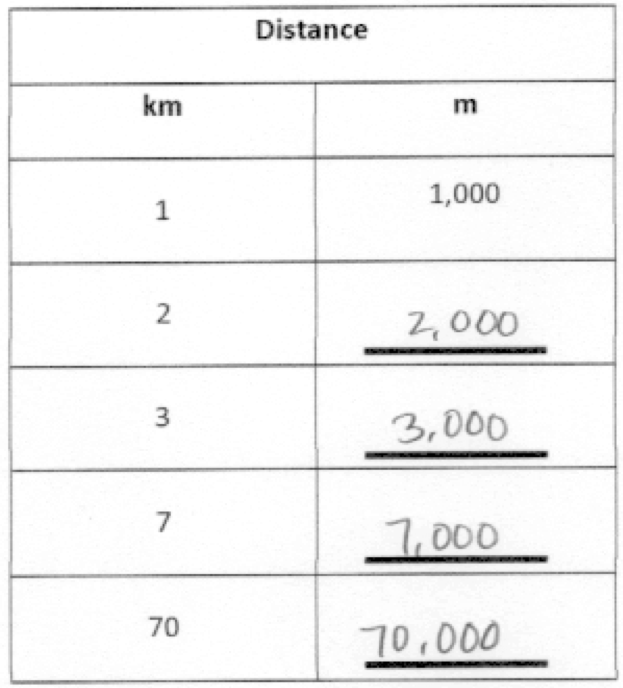Compare problems often intersect with measurement contexts. The comparison might be additive or multiplicative or both. In the following task, students have to decide on a common comparison unit. Using inches, students convert from a larger unit to a smaller unit. Students could also convert from a smaller unit to a larger unit, but that is a Grade 5 expectation. (5.MD.A.1)

#### Who Is the Tallest?

Mr. Liu asked the students in his fourth grade class to measure their heights. Here are some of the heights they recorded: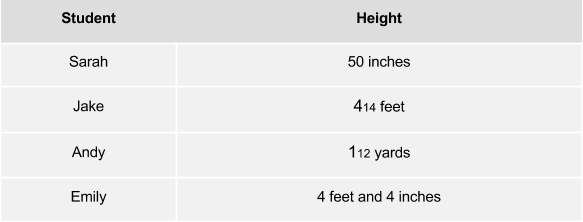List the four students from tallest to shortest.

Not only do measurement problems reinforce work with the four operations and whole numbers, but they also support operations with fractions, as illustrated in the task above and the task below. Using a number line to solve measurement problems can be a very useful strategy.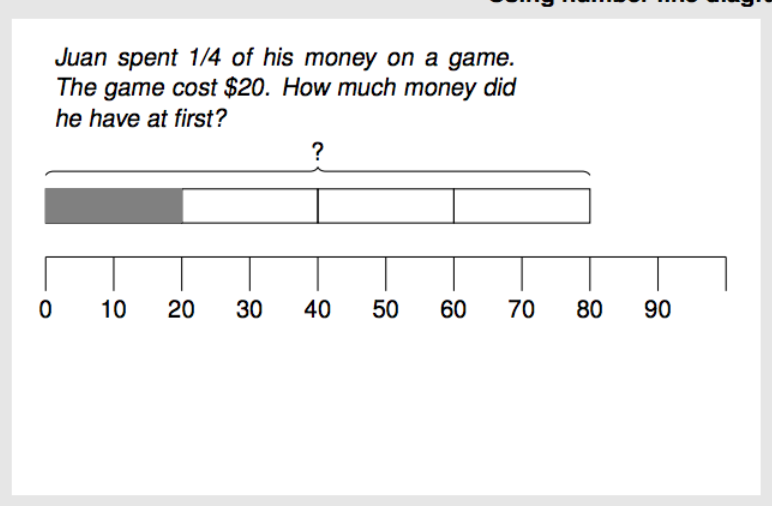## Area and perimeter

Applying the area and perimeter formulas (4.MD.A.3 )supports work with the OA standards, particularly standards in the OA.A cluster. Students were introduced to area and perimeter in Grade 3. (3.MD.C, 3.MD.D) In fact, students did a lot of work with area to support understanding of multiplication and division. However, area and perimeter formulas are new to Grade 4. Its important to note that understanding and applying formulas in context is different than writing down a memorized formula and evaluating the formula by substituting variables with known numbers. At this level, the formulas are rooted in visualizations of rectangles and use of specific numbers, not variables. The idea is that students represent these situations with equations constructed through reasoning. This way, evaluation of formulas in later grades is supported by a conceptual foundation.13 Progressions for the Common Core State Standards in Mathematics (draft): K–5, Geometric Measurement, p. 22. Progressions for the Common Core State Standards in Mathematics (draft): K–5, Geometric Measurement, p. 22. Progressions for the Common Core State Standards in Mathematics (draft): K–5, Geometric Measurement, p. 22. The example below incorporates both perimeter and multiplicative comparison.

#### Grade 4, Module 3, Lesson 3: Concept Development

Problem 1

The rectangular projection screen in the school auditorium is 5 times as long and 5 times as wide as the rectangular screen in the library. The screen in the library is 4 feet long with a perimeter of 14 feet. What is the perimeter of the screen in the auditorium?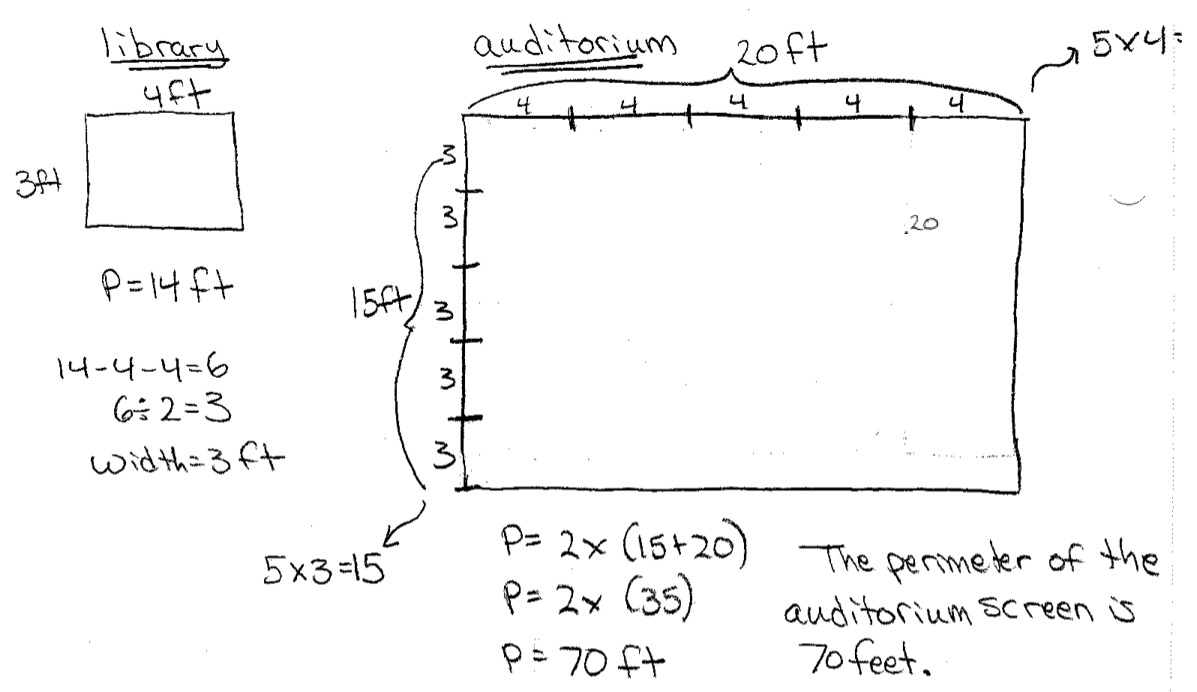The structure of this problem and what it demands of students is similar to that found within the first and second lessons of this module. Elicit from students why both the length and the width were multiplied by 5 to find the dimensions of the larger screen. Students use the dimensions to find the perimeter of the larger screen. Look for students to use formulas for perimeter other than 2 × (l + w) for this problem, such as the formula 2l + 2w.

Notice the student work does not contain a formula with variables that should be substituted. Instead students reason that the perimeter of the larger screen is twice the measure of the sum of one side length and one side width.

# Part 3: Where do Operations & Algebraic Thinking come from, and where are they going?

Podcast clip: Importance of Coherence with Andrew Chen and Peter Coe (start 9:34, end 26:19)

## Where does work with multiplication and division in Grade 4 come from?

### Grade 2: Prerequisites to multiplication

The Standards lay out a purposeful progression for multiplication and division with whole numbers. Though students begin formal study of multiplication and division in Grade 3, there are foundational skills and experiences presented in Grade 2. None of the Grade 2 standards named below are part of the major work, but they are nonetheless opportunities for students to lay the foundation for later work with multiplication; we can also leverage them for students that are struggling in Grade 4. They are:

• Students work with equal groups and arrays and use repeated addition to find the total. (2.OA.C.4) This means, for example, writing an equation like 5 + 5 + 5 + 5 = 20 to describe an array with four rows of five objects. Grade 2, Module 6, Lesson 6 from EngageNY includes materials that can be used to support students in this understanding.

• Students prepare for multiplication and division through skip-counting, which helps support Level 2 methods for multiplying and dividing. In Grade 2, students skip count by 5’s, 10’s, and 100’s (and by 2 when pairing with even numbers). (2.NBT.A.2) The fluency activity from EngageNY’s Grade 2, Module 3, Lesson 1 shows how skip-counting can be incorporated as part of a daily routine.

• Students partition rectangles into rows and columns of the same-size squares and count to find the total. (2.G.A.2) The lesson below shows an example of this.

#### Partitioning a Rectangle into Unit Squares

Materials

• Copies of a rectangle with edges marked (one for each student/ group, see attached blackline master)

• A straight edge tool

Actions

The teacher should guide students through these actions, as the text in this task is too complex for some second graders.

1. Draw a grid on the rectangle by connecting each mark to the one directly across from it on the opposite edge.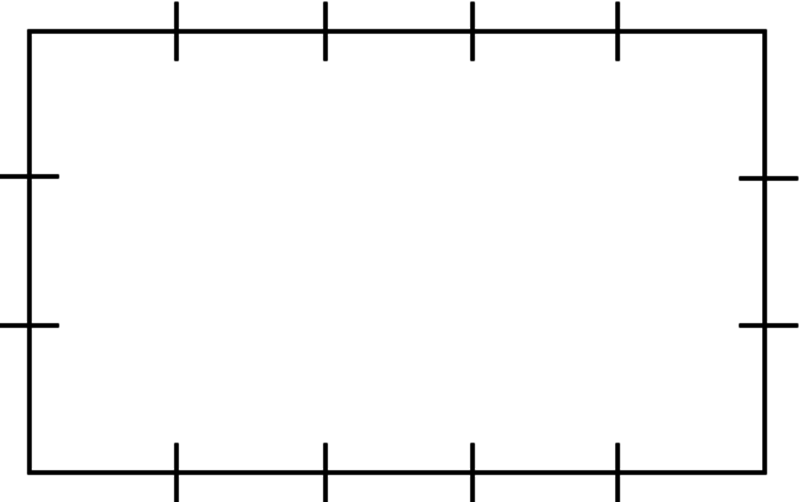1. The grid separates the rectangle into many little squares. How many squares are there?

1. There are five little squares in each row. Count by fives to find how many squares there are in the entire rectangle.

1. What other methods can you think of to quickly count how many squares there are in the entire rectangle?

1. Write a number in each little square to count them and show that your answers are correct.

1. One number sentence which shows the total number of squares is 3 + 3 + 3 + 3 + 3 = 15. Write another number sentence which shows the total number of squares.

HideShow

Multiplication is introduced in Grade 3. Here are the highlights that will be most relevant to the priority work within 4.OA:

• Students develop an initial understanding of multiplication and division by interpreting products and quotients using equal groups and arrays. (3.OA.A.1)
• Students develop a variety of strategies (including many based on properties of operations (3.OA.B.5) and the relationship between multiplication and division (3.OA.B.6)) to build toward fluency with multiplication and division within 100. (3.OA.C.7)
• Students engage in problem-solving within 100 in situations involving equal groups and arrays (3.OA.A.3) and whole number, two-step problems using the four operations. (3.OA.D.8)

Let’s take a closer look at each of these.

### Grade 3: the meaning of multiplication

Students’ initial exposure to multiplication is based on equal groups and arrays. With respect to equal groups they come to understand the meaning of the factors in equal groups situations (one factor represents the number of objects in a group and the other factor represents the number of groups) and interpret products as the total number of objects when one number is multiplied by another number. (3.OA.A.1 )In the example below, students attend to the meaning of the factors and the product. The groups are shown as bunches of flowers and there are 5 flowers in each group. The product, or total, is the result of multiplying 4 x 5.

#### Grade 3, Module 1, Lesson 3: Problem Set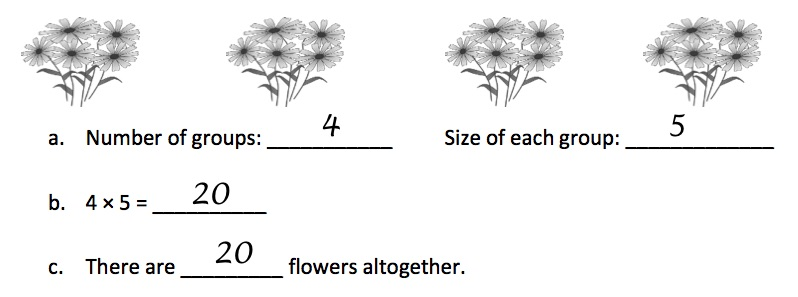Similarly, with division, students interpret quotients as either the number of shares or the number of objects in each share when a whole number of objects is partitioned equally. (3.OA.A.2)

Perhaps the most important goal for students in Grade 3 is to fluently multiply and divide within 100, knowing all multiplication facts from memory. (3.OA.C.7) To reach this goal, students develop and use strategies based on the properties of operations (the commutative and associative properties of multiplication and the distributive property (3.OA.B.5)), and the relationship between multiplication and division. (3.OA.B.6 )

The associative property of multiplication tells us that we can choose any grouping of factors and get the same product; for example, (2 x 3) x 4 and 2 x (3 x 4) both yield 24. How does this help with multiplication? For two numbers being multiplied, students can use the associative property, after decomposing one of the numbers multiplicatively into its factors, to make an easier multiplication fact. For example, when multiplying 16 x 3, a student might decompose 16 into 8 x 2 and use the associative property to make an easier fact of 8 x 6:

16 x 3 = (8 x 2) x 3 = 8 x (2 x 3) = 8 x 6 = 48

The understanding behind the associative property of multiplication should be developed conceptually, using concrete and/or pictorial representations. An example of a lesson that does this can be found here: Grade 3, Module 3, Lesson 9.

The distributive property relates multiplication and addition; for example, both 2 x (3 + 4) and 2 x 3 + 2 x 4 result in 14. Similar to what we just looked at with the associative property, students can also make an easier multiplication problem by decomposing one of the factors into its addends (additive decomposition) and then use the distributive property. Again, we can build conceptual understanding of this property through the use of visuals. The following example comes from an EngageNY lesson on the distributive property. In the concept development part of the lesson, students use the 5 + n pattern to decompose a factor into its addends (i.e., the numbers 6, 7, 8, and 9 can be thought of as 5 + 1, 5 + 2, 5 + 3, and 5 + 4 respectively). Then they multiply the easier facts and find the sum. In the homework problem below, the 8 is decomposed into 5 + 3 because knowing facts of 5 (i.e., 5 x 4) and 3 (i.e., 3 x 4) is easier for students.

#### Grade 3, Module 1, Lesson 16: Homework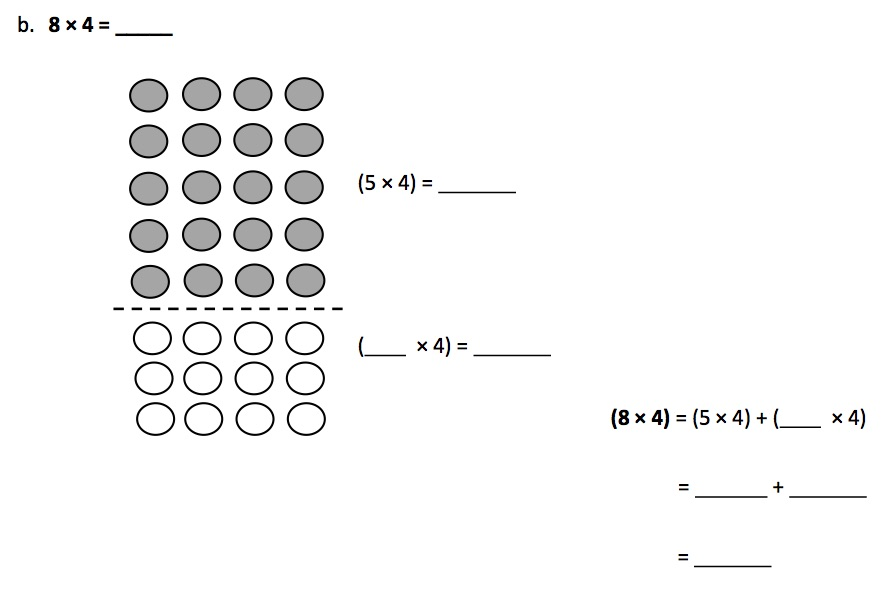The distributive property is introduced and used when multiplying single-digit numbers to help students strategically progress toward fluency with the more challenging facts. For example, instead of rote memorization of 8 x 4, students can use the distributive property to multiply easier facts that they may be more fluent with, like (4 x 4) + (4 x 4) or (2 x 4) + (6 x 4). Students continue to use these properties as they move on to multiplying single-digit numbers by 2-digit numbers (with products within 100 in Grade 3). In later grades, the distributive property is at the root of understanding the standard algorithm for multiplication.

The commutative property of multiplication lets us know we can change the order of the factors in a multiplication expression; for example, 2 x 3 and 3 x 2 both result in 6. The commutative property helps students access a wider range of facts; for example, if students know 9 x 6 then they also know 6 x 9. It should be introduced conceptually. The following lesson from EngageNY introduces students to the commutative property of multiplication by using an array and then rotating the array 90 degrees. As observed, the product is the same, however, the position of the factors changed.

#### Grade 3, Module 1, Lesson 7: Concept Development

Concept Development (32 minutes)

Materials: (S) Personal white board

Problem 1: Rotate arrays 90 degrees.

T: Position your board so that the long side is horizontal. Draw an array that shows 4 rows of 2.

S: (Draw the array, as shown here)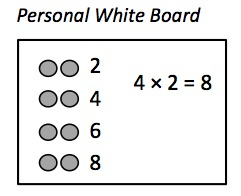T: Write a skip-count by twos to find the total. Then write a multiplication sentence where the first factor represents the number of rows.

S: (Write 2, 4, 6, 8 and 4 × 2 = 8 as shown)

T: Rotate your board 90 degrees so that the long side is vertical.

S: (Rotate, as shown here.)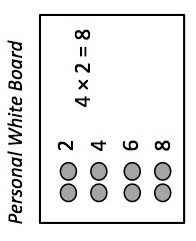T: What happened to the array?

S: It has 2 rows of 4. → It has 4 groups of 2, but they’re up and down instead of in rows.

T: Now the twos are columns, vertical groups in an array.

T: I’ll rotate my board. You tell me if the twos are columns or rows.

T: (Show the twos as rows.)

S: Rows!

T: (Rotate your board and show the twos as columns.)

S: Columns!

T: Skip-count the rows by four!

S: (Point to the rows as students count.) 4, 8.

T: Add that skip-count to your board. (Allow time.) What multiplication sentence can represent this array?

S: 2 × 4 = 8.

T: (Write 4 × 2 = 8 and 2 × 4 = 8 on the board with their corresponding arrays drawn as shown.) What do you notice about the multiplication sentences?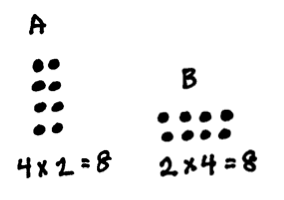S: The 4 and the 2 switched places.

T: What do the 4 and 2 represent in each? Talk to your partner.

S: In A, the 4 represents the number of rows, but in B, it represents the size of the row. → The twos are rows in A but columns in B.

T: Did the meaning of the 8 change?

S: No.

T: So factors can switch places and trade meanings, but the total stays the same. We call that the commutative property. Talk to your partner about why the total stays the same.

S: (Discuss.)

Continue with 2 × 5 and 3 × 4 arrays.

HideShow

The commutative property helps students recognize equivalence of multiplication facts. In the lesson above, 4 rows of 2 and 2 rows of 4 have the same product. Thus, 4 x 2 = 2 x 4. Students can apply the commutative property as a strategy for knowing more multiplication facts. If students know 4 x 2 then they also know 2 x 4. This is especially useful when students are working toward fluency with the more challenging single-digit numbers.

Consistent, sustained work developing and applying the properties above will lead students to meet the Grade 3 fluency goals. The following fluency mini-assessment, developed by Student Achievement Partners, assesses fluency with two one-digit numbers and is an illustration of the kind of fluency work students do in Grade 3. The unknowns are placed in all positions to emphasize the relationship between factors and quotients.

#### Multiplication and Division Within 100 Mini-AssessmentIn Grade 3, students use multiplication and division to solve word problems; they also extend problem-solving from K-2 to include two-step problems using the four operations. Let’s take a closer look at each of these.

Students use multiplication and division within 100 to solve word problems in situations involving equal groups, arrays, and measurement quantities. (3.OA.A.3) These situations are described in the first two rows of Table 2.15 CCSSM Table 2. CCSSM Table 2. CCSSM Table 2. The task below uses the context of money (work with whole dollar amounts begins in grade 2 (2.MD.C.8)).

#### Gifts From Grandma, Variation 1

1. Juanita spent \$9 on each of her 6 grandchildren at the fair. How much money did she spend?
2. Nita bought some games for her grandchildren for \$8 each. If she spent a total of \$48, how many games did Nita buy?
3. Helen spent an equal amount of money on each of her 7 grandchildren at the fair. If she spent a total of \$42, how much did each grandchild get?

Solutions

Solution: Tape diagram

This task needs a tape diagram solution.

Solution: Writing multiplication equations for division problems

1. Sandra spent 6 groups of \$9, which is 6 × 9 = 54 dollars all together.
2. Since the number of games represent the number of groups, but we don’t know how many games she bought, this is a "How many groups?" division problem. We can represent it as

? × 8 = 48

or

48 ÷ 8 = ?

So Nita must have bought 6 games.

1. Here we know how many grandchildren there are (so we know the number of groups), but we don’t know how much money each one gets (the number of dollars in each group). So this is a "How many in each group?" division problem. We can represent it as

7 × ? = 42

or

42 ÷ 7 = ?

So Helen must have given each grandchild \$6.

HideShow

The task shows three equal groups problems in which the unknown is in a different position. The first problem is a multiplication problem in which the product is unknown (Unknown Product). The next two problems show two related division/unknown factor problems: "How many groups?" (Number of Groups Unknown) and "How many in each group?" (Group Size Unknown).

Also in Grade 3, students solve two-step word problems involving any combination of the four operations. In addition, formal use of algebraic language begins in Grade 3 with use of a letter for unknown. While students have been using a symbol for the unknown since first grade, specifically using a letter for the unknown begins in Grade 3. Two-step problems may require one representation and solution or more difficult problems may require two representations and solutions. The task below illustrates a multiplication and subtraction problem in which the solution is shown in two steps and an equation using a letter for the unknown is used to represent the problem.

#### The Class Trip

Mrs. Moore’s third grade class wants to go on a field trip to the science museum.

• The cost of the trip is \$245.
• The class can earn money by running the school store for 6 weeks.
• The students can earn \$15 each week if they run the store.

1. How much more money does the third grade class still need to earn to pay for their trip?

1. Write an equation to represent this situation.

Solution

1. We can start by finding out how much money the students can make at the store: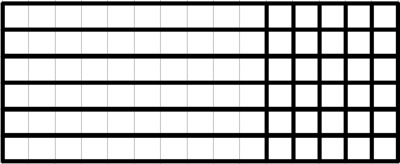6 × 15 = 6 × 10 + 6 × 5 = 60 + 30 = 90

Since

245 − 90 = 155

the students still need \$155 dollars for the field trip.

1. We can let n stand for the amount of money they still need. We know that the amount they can make at the store is 6×15 and the amount they need to raise is 245, so one equation is

245 − 6 × 15 = n

Another possible equation is

6 × 15 + n = 245

In the example above, the solution shows the use of an area model to multiply 6 x 15 and shows an equation to subtract 245 – 90. Then students reason about the solution steps (i.e., multiplication and subtraction) in order to represent the entire problem with one equation. In Grade 3, students use area contexts to support understanding of multiplication and division. (3.MD.C.7) Students relate arrays to rectangular areas and use this relationship to show that multiplying the side lengths of a rectangle yields the area of the rectangle. From there, students can use area models to represent multiplication problems.

### Suggestions for students who are below grade level

So what do all of these prerequisites mean? If students come to Grade 4 without a solid grasp of the ideas named above (or haven’t encountered them at all), what can you do? It’s not practical (or even desirable) to reteach everything students should have learned in Grades 2-3; there’s plenty of new material in Grade 4, so the focus needs to be on grade-level standards. At the same time, there are strategic ways of wrapping up “unfinished learning” from prior grades. Here are a few suggestions for adapting your instruction to bridge the gaps with respect to preparedness for multiplication and division with whole numbers.

• If a significant number of your students lack the skill and understanding of the Grade 2 prerequisites for multiplication, work with visual models (such as arrays and partitioning rectangles) and skip-counting may offer necessary scaffolding. Planning a few introductory lessons that ask students to analyze and write repeated addition equations from arrays or tiled rectangles to begin a unit on multiplication and division can support students with these prerequisites. This module provides lessons that introduce students to arrays (Topic B) and partitioning rectangles (Topic C). For skip-counting, consider a daily fluency warm-up that gives students time to learn and practice this skill, such as the warm-up activity in this lesson.
• If a significant number of your students are struggling with interpreting products as the total number of objects in equal groups and solving word problems involving arrays and/or equal groups, consider planning an introductory lesson on these Grade 3 understandings. This lesson is a good place to start. Once this idea is introduced, a “problem of the day” or warm-up structure using, for example, the application problems found throughout this module will help students with this prerequisite understanding. Simpler two-step word problems like this one may also be appropriate for students that are struggling with grade level problems using the four operations. A daily two problem sequence featuring one problem from Grade 3 and one from Grade 4 can be an effective strategy for building to grade level work.
• If a significant number of your students lack fluency with multiplication and division, spending a few days on strategies based on the properties of multiplication at the start of a unit on multiplication and division may be well spent. This lesson on using the distributive property to multiply and this lesson on the associative property are good places to start. Once students are comfortable applying these strategies, consider incorporating daily fluency activities like multiplication and division sprints to support fluency; the “pattern sheet” activities associated with the lessons in this module are examples of these.

## Beyond Grade 4: What’s next with multiplication and division?

It is helpful to understand how students will build on their understanding of multiplication and division in later grades. Understanding the progression of this content can help to solidify the focus in fourth grade. It helps us to define the limits of our instruction in Grade 4 and helps us see why we focus so intently on a small number of concepts. At the same time, we realize that we want to set our students up for future success, and knowing the next steps in their journey can help us focus our lessons on the knowledge and skills that matter most.

In Grade 5, students extend their understanding of multiplicative comparisons to interpret multiplication as scaling, including the use of fractions.5.NFB.B.3 In the example below, students use multiplicative comparison, with a context including fractions, to scale a quantity up.

Students are also expected to fluently use the standard algorithm for multiplication by the end of Grade 5 (5.NBT.B.5 )and by end of Grade 6 for division. (6.NS.B.2 )Students use visuals like area models to provide meaning for algorithms. The example below shows how a student might support multi-digit multiplication using an area model and connecting that area model to the standard algorithm.

#### Grade 5, Module 2, Lesson 6: Concept Development

Problems 2–3

814 × 39

624 × 82

T: (Write 814 × 39 on the board.) Partner A, use the standard algorithm to solve. Partner B, draw an area model to solve.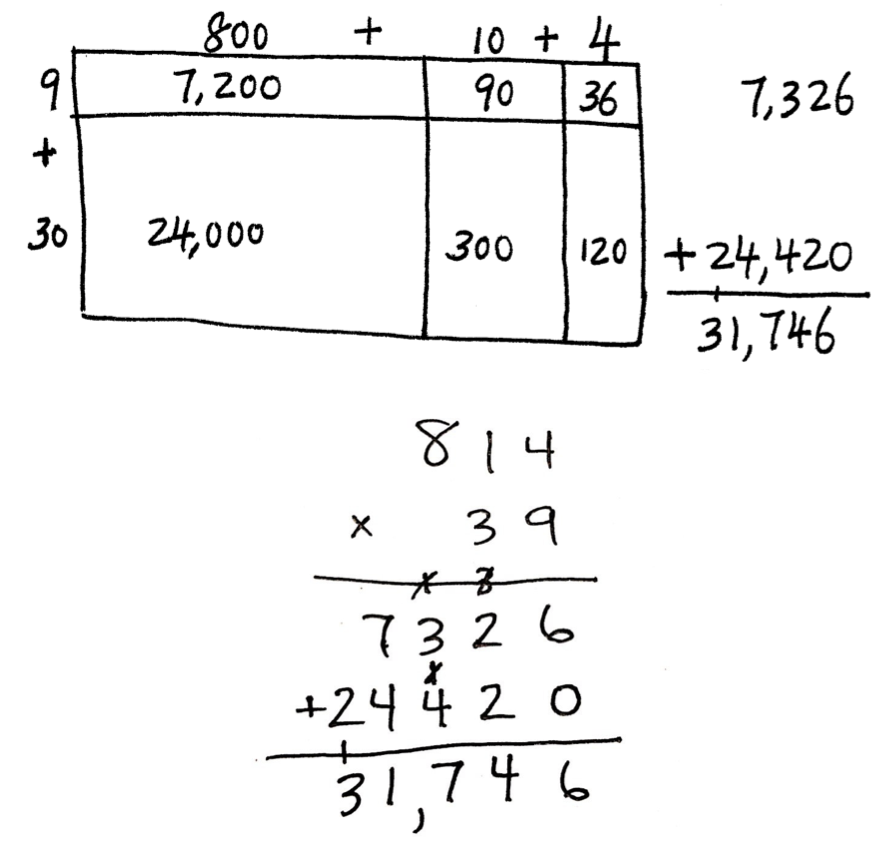T: (Draw and solve.)

Examples like these are an important part of work in Grade 5; students become comfortable with the standard algorithm by connecting it to prior work with visual models and other strategies.

Student Achievement Partners: Focus in Grade 4

Draft K-5 Progression on Counting and Cardinality and Operations and Algebraic Thinking

EngageNY: Grade 4 Module 3 Materials

# Endnotes

 The Common Core State Standards for Mathematics (CCSSM) are organized into major, additional and supporting clusters in the Focus by Grade Level documents from Student Achievement Partners.

 Progressions for the Common Core State Standards in Mathematics (draft): K, Counting and Cardinality; K–5, Operations and Algebraic Thinking, p. 29.

 Adapted from CCSSM Table 2.

 For more information about these properties see “Properties of multiplication and the relationship between multiplication and division” in the UnboundEd Grade 3 Guide to the Operations & Algebraic Thinking domain.

 Decomposing and composing numbers to make easier multiplication and division problems is considered a Level 3 multiplication method. This is the most sophisticated strategy used by students in working toward fluency with single-digit facts in Grade 3. The Level 1 method is representing and counting all. The Level 2 method is counting on by (i.e., skip-counting). The Level 3 method rests on understanding of the associative property of multiplication and the distributive property and both properties require decomposition of numbers. You can read more about how students use Level 3 methods of computation to establish fluency with multiplication and division in the Draft K–5 Progression on Counting and Cardinality and Operations and Algebraic Thinking or the UnboundEd Grade 3 Guide to the Operations & Algebraic Thinking domain.

 In Grades K-2, students focus extensively on representing and solving word problems involving addition and subtraction of whole numbers within 100. Table 1 from CCSSM describes the different problem-solving situations students are expected to master by end of Grade 2. Students are expected to continue to engage with these problem-solving situations in Grades 3-5, however, students use larger numbers, numbers other than whole numbers, and combine the use of several operations to solve multistep word problems. It is important to become familiar with the problem-solving situations described in Table 1.

 Front Matter for Progressions for the Common Core State Standards in Mathematics (draft), p. 12.

 From left to right the examples are taken from EngageNY: Grade K, Module 4, Lesson 3; Grade 1, Module 1, Lesson 5; Grade 2, Module 4, Lesson 2; Grade 3, Module 1, Lesson 3; Grade 3, Module 1, Lesson 18.

 Progressions for the Common Core State Standards in Mathematics (draft): K, Counting and Cardinality; K–5, Operations and Algebraic Thinking, p. 30.

 Progressions for the Common Core State Standards in Mathematics (draft): Grade 8, High School, Functions, p. 4.

 The idea that standards relate strongly to one another is known as coherence, and is a distinctive feature of the Common Core State Standards for Math. If you’re interested in exploring more of the connections between standards, you might want to check out the Student Achievement Partners Coherence Map, which illustrates them visually.

 Progressions for the Common Core State Standards in Mathematics (draft): K, Counting and Cardinality; K–5, Geometric Measurement, p. 20.

 Progressions for the Common Core State Standards in Mathematics (draft): K–5, Geometric Measurement, p. 22.

This is not the first time students have engaged with properties of operations. Underlying students work with addition and subtraction in Grades K-2 is an understanding of the properties of addition, as well as knowledge of the relationship between addition and subtraction, and fluidity with decomposing and composing numbers in order to add and subtract. (K.OA.A.3, 1.OA.C.6) Students apply these understandings, in addition to place value understandings, as strategies to solve increasingly complex addition and subtraction problems.

 CCSSM Table 2.

# FAQs

## 1. What is a Content Guide?

Our goal in creating the Content Guides has been to provide busy teachers with a practical and easy-to-read resource on what the grade-level math standards are saying, along with examples of instructional materials that support conceptual understanding, problem-solving, and procedural skill and fluency for students.

It’s important to note that content guides are not meant to serve as a curriculum (or any kind of student-facing document), a guide or source material for test-preparation activities, or any kind of teacher evaluation tool.

## 2. What’s in a Content Guide?

Each Content Guide is focused on a specific group of standards. Most Content Guides follow the same three-part structure:

• Part 1 makes clear the student skills and understandings described by this group of standards. This section illustrates the standards using multiple student tasks from freely available online sources. Teachers can use or adapt these tasks for their students.
• Part 2 explains how this group of standards is connected to other standards in the same grade. We highlight how these connections have implications for planning and teaching, and how this within-grade coherence can increase access for students. Part 2 also includes multiple student tasks from freely available online sources.

• Part 3 traces selected progressions of learning leading to grade-level content discussed in the specific Content Guide. This discussion segues into a series of concrete and practical suggestions for how teachers can leverage the progressions to teach students who may not be prepared for grade-level mathematics. Finally, Part 3 traces the progression to content in higher grades.

## 3. How can I use the Content Guides?

Teachers who have read our Content Guides say they see benefits for all educators. Here are some suggestions for how different educators might use them.

Teachers can use the Mathematics Content Guides to:

• Increase or refresh their knowledge of the standards and the expectations for what students should know by the end of the year.
• Adapt lessons and units using appropriate pre-requisites to support students who are behind grade-level.
• Gain access to the best available OER for math to use for introducing and/or reinforcing concepts
• Ensure their curriculum and/or units:
• Focus on the major work of the grade and the appropriate depth of each standard.
• Target the appropriate aspects of rigor—procedural skill and fluency, modeling and application, and conceptual understanding described by the standards.
• Help students make coherent connections within and across grades.
• Create or revise their lessons and questioning to focus on important concepts in the standards.

Instructional coaches and school leaders can use the Mathematics Content Guides to:

• Refresh or increase their knowledge of the standards and the expectations for what students should know by the end of the year.
• Develop and communicate consistent expectations for lesson planning and instruction aligned to the standards.
• Provide a reference when planning and/or discussing instruction with teachers.
• Gain insight into what instruction and student work should look like in order to meet the demands of the standards.
• Develop and design content and standards-driven professional development sessions/workshops.
• Foster content rich, standards-based discussions among staff and build staff knowledge.
• Develop and/or revise school improvement plans in order to support and incorporate content and practice-based teaching and learning.

## 4. Why the Content Guides?

The transition to higher standards has led teachers all over the country to make significant changes in their planning and instruction, but only one-third of teachers feel they are prepared to help their students pass the more rigorous standards-aligned assessments (Kane et.al., 2016). This is to be expected because the new high standards are a significant departure from prior standards. The standards require a deeper level of understanding of the math content they teach; a different progression of what students need to learn by which grade; as well as different pedagogy that emphasizes student conceptual understanding, problem solving and procedural fluency in equal intensity.

The support for teachers to bring high standards to their classrooms, however, has lagged behind. Research shows that teacher training in the U.S. is currently insufficient in preparing teachers to teach the demanding new standards (Center for Research in Mathematics and Science Education, 2010). And though some resources exist that “unpack” the standards, few, if any, explain and illustrate the standards. “Unpacking” the standards one by one can also result in a disjointed presentation that neglects the structure and coherence of the standards. In creating the Content Guides, we aimed to provide busy teachers with a practical, easy-to-read resource on their grade-specific standards and how to help all students learn them. There is ample empirical evidence that when teachers have both strong knowledge of the math content that they teach, and the pedagogical knowledge to help students master that content knowledge, their students learn more (Baumert et. al., 2010; Hill, Rowan and Ball, 2005; Rockoff et. al., 2008). With the Content Guides in hand, we hope that teachers will find more success in helping their students make progress toward college- and career-readiness.

## 5. What is the relationship between the Content Guides and the Progressions?

The Progressions documents describe the grade-to-grade development of understanding of mathematics. These were informed by research on children’s cognitive development as well as the logical structure of mathematics. The Progressions explain why standards are sequenced the way they are. The Content Guides often highlight key ideas from the Progressions, but do not add new standards or change the expectations of what students should know and be able to do; they aim to explain and illustrate a group of standards at a time using freely available online sources. While the OER tasks and lessons in the Content Guides are one way to meet the grade-level standards, they are not the only means for doing so.

## 6. How were the resources selected?

We selected sample tasks and lessons from freely available online sources such as EngageNY, Illustrative Mathematics and Student Achievement Partners to illustrate the Standards. These sources are chosen because they are fully aligned to the new high standards based on national review of K-12 curricula or are created by organizations led by the writers of the new high standards. In addition, because they are open educational resources (OER), they are freely accessible for all uses. All UnboundEd materials are also OER, as part of our commitment to make high-quality, highly aligned content available to all educators.